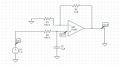# Opamp output voltage

#### liviu93

Joined Nov 15, 2015
1
Hello to all,
I have the following circuitand I need to find.
Because this is a non-inverting opamp=(R2/R1 + 1).
https://en.wikipedia.org/wiki/RC_circuit#Parallel_circuit says :
"the output voltageis equal to the input voltage" .
So, Uin = Uin+.
is that correct?

#### Jony130

Joined Feb 17, 2009
5,456
Yes and No, it all depend on input signal frequency. If input signal is a DC voltage (F = 0Hz) than yes Uin = Uin+
But for example if input signal has a frequency equal to F = 1/(2 * pi *R3 * C2) ≈ 0.16/(R3 *C2) = 0.16/(1kΩ*1μF) = 160Hz then Uin+ = 0.707*Uin Because this RC circuit at the input act just like a low pass filter - voltage divider whose attenuation (gain) depends on the input signal frequency.
https://en.wikipedia.org/wiki/RC_circuit#Series_circuit

•liviu93

#### hsazerty2

Joined Sep 25, 2015
30
Because this is a non-inverting opamp=(R2/R1 + 1).
This is a non-inverting amplifier, so that's right, we have:

Uout=(Uin+)*(1+R2/R1)

Next is to find a relation between Uin+ and V2; It's an RC circuit, with the capacitor charging from 0v (if we assume zero initial condition like i guess) to 1V. The equation is then:

Uin+=(V2)*(1-Exp(-t/(R*C)))

So finally:

Uout=(1+R2/R1)*(V2)*(1-Exp(-t/(R*C)))

I guess that's it.

#### bertus

Joined Apr 5, 2008
22,215
Hello,

@hsazerty2
In this home work forum, you do not give complete solutions, but hints to get to the solution.
The topic starter will not learn much from complete solutions.
When they have to do some work on their own, the learned stuff will be better understanded.

Bertus

#### hsazerty2

Joined Sep 25, 2015
30